Math Calculators, Lessons and Formulas

It is time to solve your math problem

mathportal.org
• Geometry
• Hexagonal pyramid

# Hexagonal pyramid

ans:
syntax error
C
DEL
ANS
±
(
)
÷
×
7
8
9
4
5
6
+
1
2
3
=
0
.
auto next question
calculator
•  Question 1: 1 pts A hexagonal pyramid has 7 faces, 6 of which are triangles and one which is a hexagon.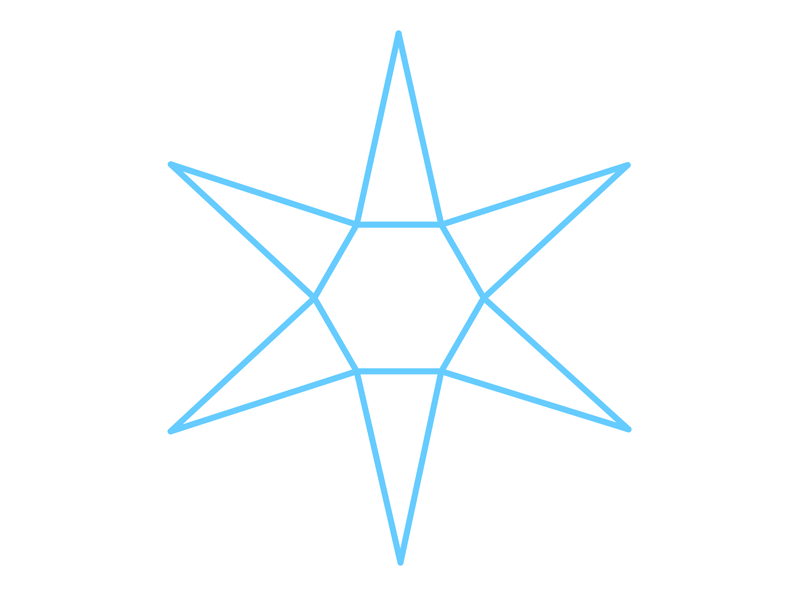•  Question 2: 1 pts How can we calculate the base area of a hexagonal pyramid?
 $A=3\cdot \dfrac{a^{2}\sqrt{3}}{4}$ $A= \dfrac{a^{2}\sqrt{3}}{2}$ $A=6\cdot \dfrac{a^{2}\sqrt{3}}{2}$ $A=3\cdot \dfrac{a^{2}\sqrt{3}}{2}$
•  Question 3: 1 pts Find the length of the lateral height of the regular hexagonal pyramid shown on the picture.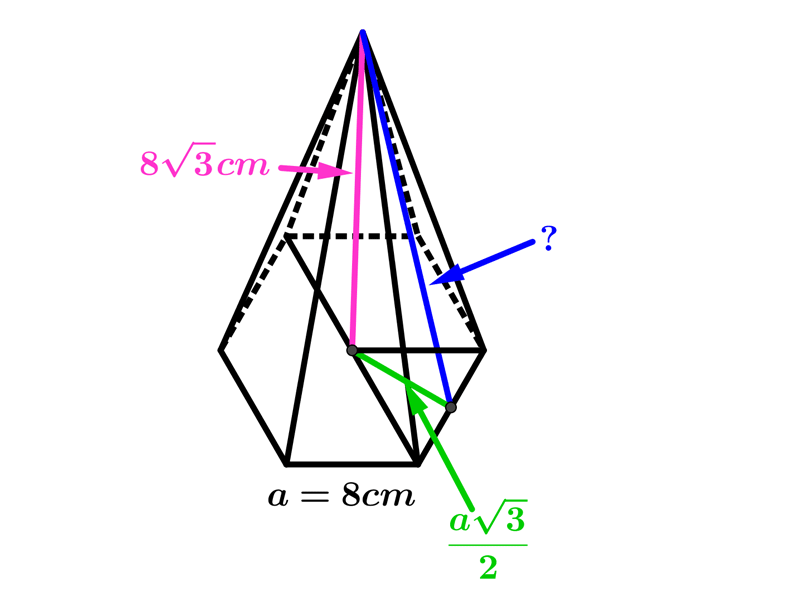$9\sqrt{5}cm$ $4\sqrt{15}cm$ $5\sqrt{17}cm$ $4\sqrt{3}cm$
•  Question 4: 1 pts Find the length of the height of the regular hexagonal pyramid shown on the picture.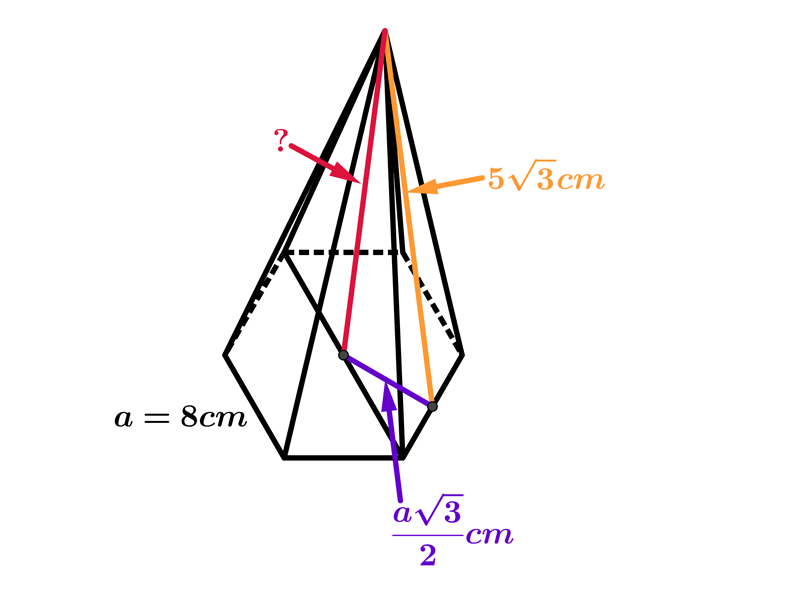$9\sqrt{3}cm$ $4\sqrt{5}cm$ $3\sqrt{3}cm$ $4\sqrt{3}cm$
•  Question 5: 2 pts The basic edge of the regular hexagonal pyramid is 6 cm, and the height of the pyramid is equal to the shorter diagonal of the base. Find the volume of the pyramid. *shorter diagonal $=a\sqrt{3}$
 $\dfrac{1}{4}\cdot 6\cdot \dfrac{6^{2}\sqrt{3}}{2}\cdot 6\sqrt{3}$ $\dfrac{1}{3}\cdot 6\cdot \dfrac{6^{2}\sqrt{3}}{4}\cdot 6\sqrt{3}$ $\dfrac{1}{3}\cdot 6\cdot \dfrac{6\sqrt{3}}{4}\cdot 6\sqrt{3}$ $\dfrac{1}{3}\cdot 6\cdot \dfrac{6^{2}}{4}\cdot 12$
•  Question 6: 2 pts The slant edge of a right regular hexagonal pyramid is $10 cm$ and the height is $8cm.$ Find the area of the base.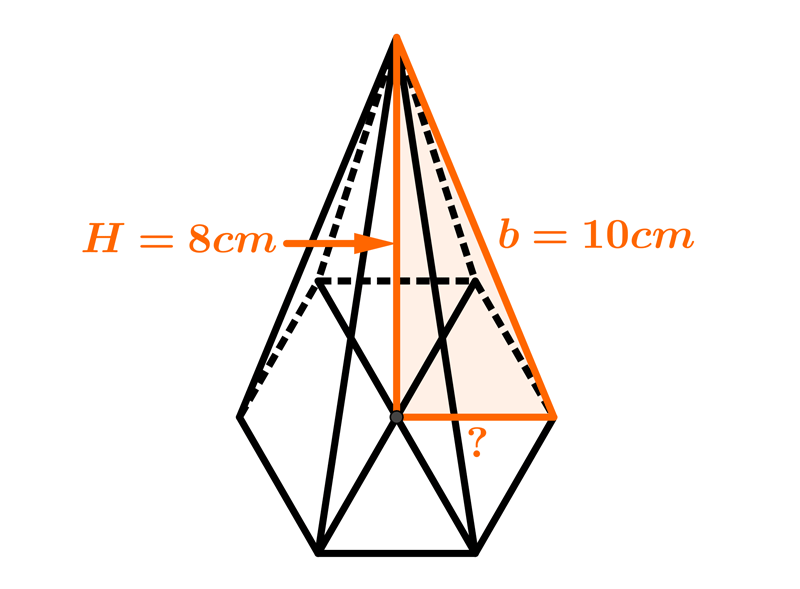Area of the base$=$
•  Question 7: 2 pts The slant edge of a right regular hexagonal pyramid is $b=3\sqrt{5}cm$ and the base edge is $6cm.$ Find the volume of that pyramid.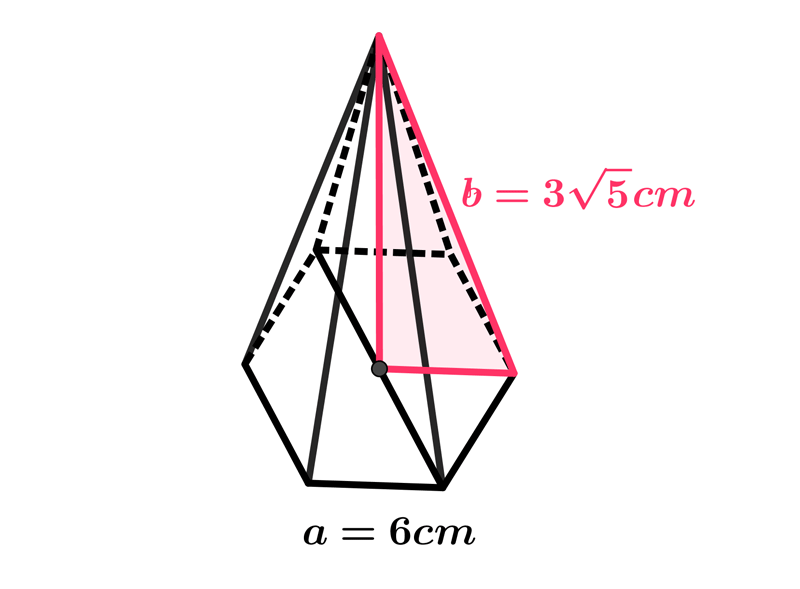$48\sqrt{3}cm^{3}$ $49\sqrt{3}cm^{3}$ $54\sqrt{3}cm^{3}$
•  Question 8: 2 pts A regular hexagonal pyramid has the perimeter of its base $24cm$ and its altitude is $15cm.$ Find its volume.
 $81\sqrt{3}cm^{3}$ $96\sqrt{3}cm^{3}$ $108\sqrt{3}cm^{3}$ $120\sqrt{3}cm^{3}$
•  Question 9: 3 pts Find the surface area of a regular hexagonal pyramid whose height is $6cm$ and the radius of a circle inscribed in the base is $2\sqrt{3}cm.$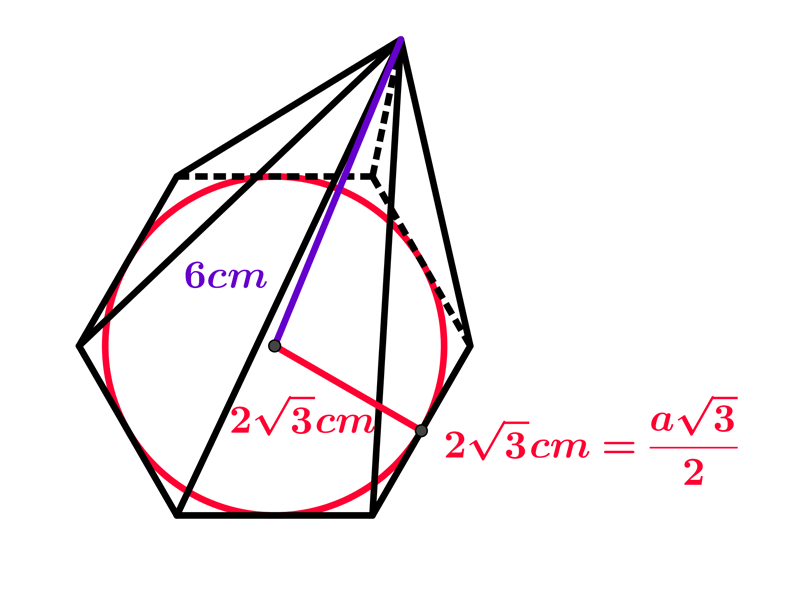Surface area $=$
•  Question 10: 3 pts The lateral surface area of regular hexagonal pyramid is $108cm^{2},$ a the area of its base is $54\sqrt{3}cm^{2].$ Find the volume of that pyramid.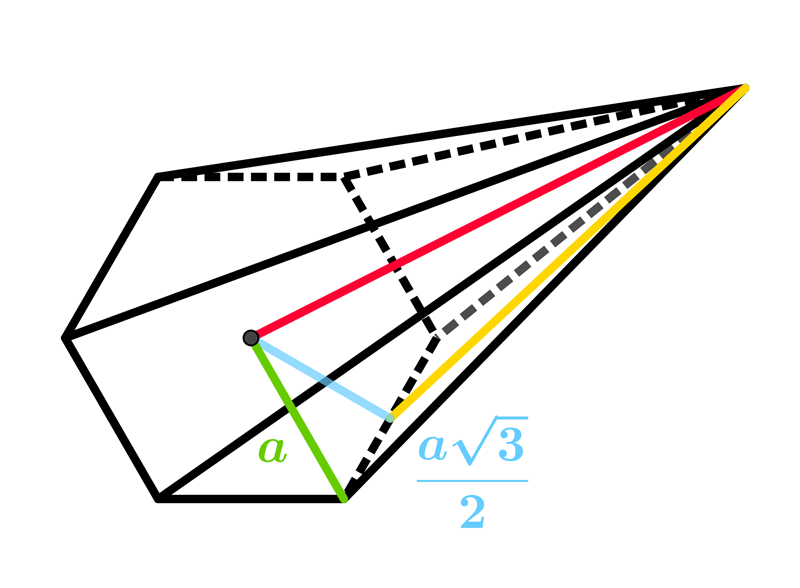$V=54\sqrt{3}cm^{3}$ $V=108\sqrt{3}cm^{3}$ $V=162\sqrt{3}cm^{3}$
•  Question 11: 3 pts The base of a right pyramid is a regular hexagon of side $8cm$ and its slant surfaces are inclined to the horizontal at an angle of $60^{\circ}$. Find the surface area.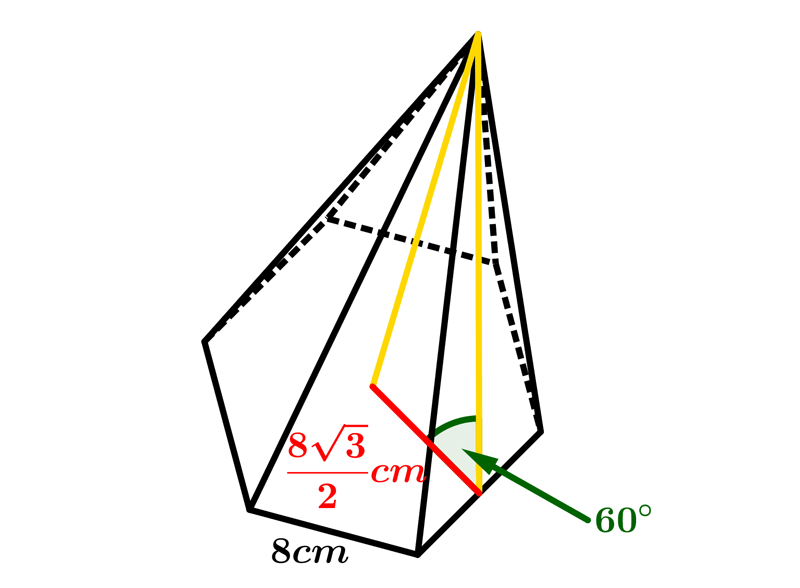Surface area $=$
•  Question 12: 3 pts Find the surface area of two pyramids with their bases stuck together.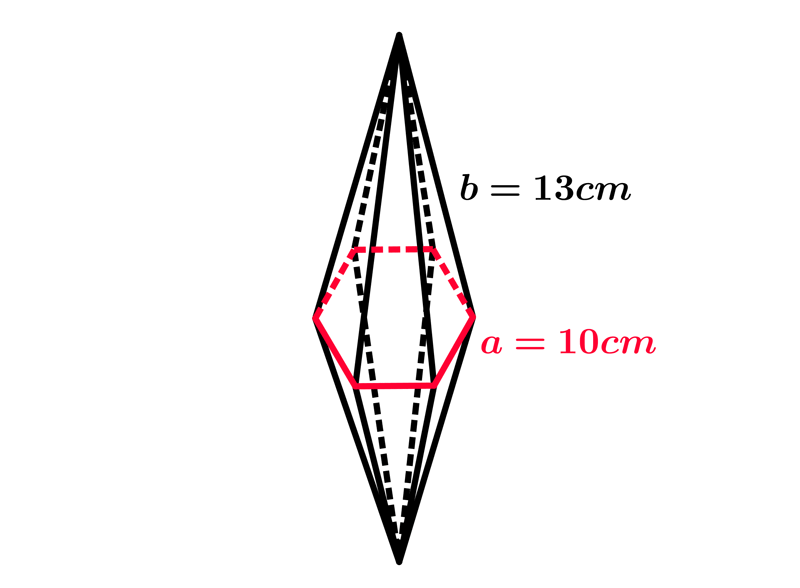Surface area $=$